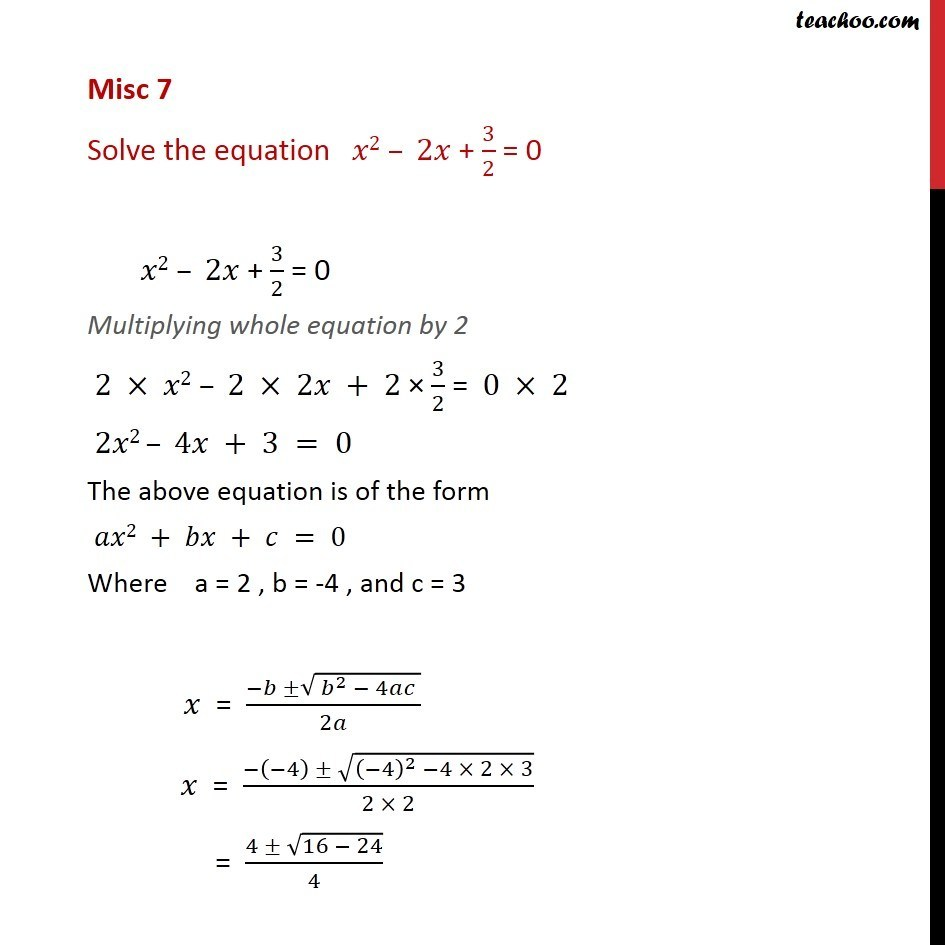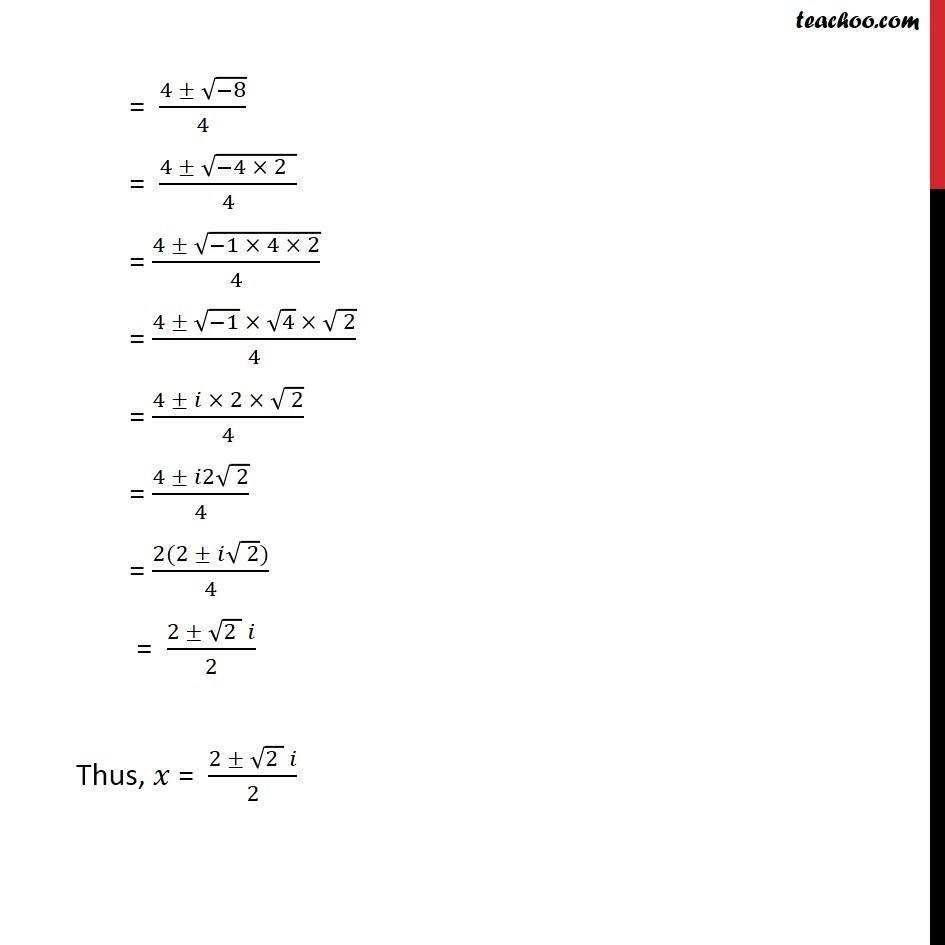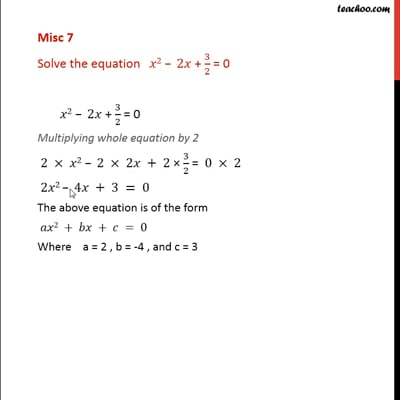Miscellaneous

Chapter 5 Class 11 Complex Numbers (Term 1)
Serial order wiseThis video is only available for Teachoo black users

### Transcript

Misc 7 Solve the equation 2 2 + 3/2 = 0 2 2 + 3/2 = 0 Multiplying whole equation by 2 2 2 2 2 + 2 3/2 = 0 2 2 2 4 + 3 = 0 The above equation is of the form 2 + + = 0 Where a = 2 , b = -4 , and c = 3 = ( ( ^2 4 ))/2 = ( ( 4) (( 4)^2 4 2 3))/(2 2) = (4 (16 24))/4 = (4 ( 8))/4 = (4 ( 4 2 ))/4 = (4 ( 1 4 2))/4 = (4 ( 1) 4 ( 2))/4 = (4 2 ( 2))/4 = (4 2 ( 2))/4 = (2(2 ( 2)))/4 = (2 (2 ) )/2 Thus, = (2 (2 ) )/2